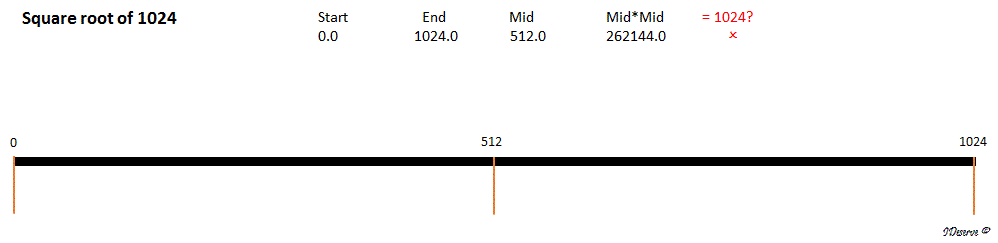# Square root of a number

Given an integer, how do you find the square root of the number without using any built-in function?
If the number is not a perfect square then take precision = 0.0005.
The number can be a positive or negative integer.

Example:
Square root of 1024 = 32
Square root of 2 = 1.41455078125
Square root of -256 = 16i (i = iota = √-1)

## Algorithm/Insights

In this algorithm, we use binary search to find the square root of the number.
It is recommended to go through Binary Search topic first.

At every step, we find out the mid value from start(initialized as 0) and end(initialized as number) and then check if it is the square root (in case of perfect square numbers) or whether we are reaching the square root within give precision by comparing current mid value to previous mid value (in case the number is not a perfect square).

Steps:
Use binary search to find the square root.
1. Initialize, start = 0, end = number, mid = (start+end)/2.
2. Set prevMid = 0, as the previous mid value.
3. Find diff = absolute difference between prevMid and mid.
4. While mid is not the square root of number (i.e. mid*mid != number) and difference diff is greater than 0.0005,
repeat the following steps:
a. If mid*mid > number, then the square root will be less than mid. So, set end = mid.
b. Else, the square root will be greater than mid. So, set start = mid.
c. Set prevMid = mid
d. Re-evaluate mid  = (start+end)/2.
e. Re-evaluate diff from prevMid and mid.

The algorithm strategy is explained with the help of following animation:If the given number is negative, then square root of the number will be square root of positive part of the number * i (iota).
For example:
-100 = √100 * √-1 = 10i

## Code Snippet

```
package com.ideserve.questions.saurabh;

/**
* <b>IDeserve <br>
* Given an integer, find the square root of the number.
* If the number is not a perfect square then take precision = 0.0005.
* The number can be a positive or negative integer.
*
* @author Saurabh
*/
public class SquareRoot {

private static String findSquareRoot(int number) {

Boolean isNegative = false;
if(number < 0) {
number = -number;
isNegative = true;
}

if(number == 1) {
return number + (isNegative ? "i" : "");
}

double start = 0;
double end = number;
double mid = (start+end)/2;
double prevMid = 0;
double diff = Math.abs(mid - prevMid);
double precision = 0.0005;

while((mid*mid != number) && (diff > precision)) {
if(mid*mid > number) {
end = mid;
} else {
start = mid;
}
prevMid = mid;
mid = (start+end)/2;
diff = Math.abs(mid - prevMid);
}

return mid + (isNegative ? "i" : "");
}

public static void main(String[] args) {
int number = 2;
System.out.println("Square root of " + number + " = " + findSquareRoot(number));
}
}
```